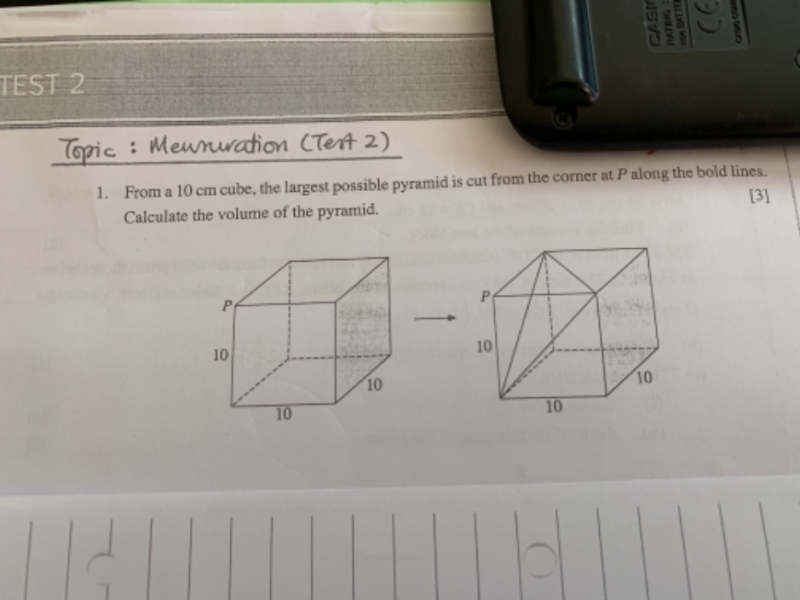# QuestionPls help. S2 math.

Thanks.

Volume of pyramid = 1/3 (height x base area)

The base is an equilateral triangle with side S = (102 + 102 )-2 = 10√2  [according to a2 + b2 = c2 ]

The area of the equilateral triangle B= {[S2 – (S/2)2 ]-2 × S }/2  [according to a2 + b2 = c2 & area = 1/2 height × base]

B = {[10√2 × 10√2 – 5√2 × 5√2 ]-2 × 10√2 }/2

= {[2 × 102 – 2 × 25]-2 × 10√2 }/2

= {[2 × 4 × 25 – 2 × 25]-2 × 10√2 }/2

= {[2 × 3 × 25]-2 × 10√2 }/2

= {5√6 × 10√2 }/2

= {100√3}/2

= 50√3

The height of the pyramid H = <(S/2)2 – {[S2 – (S/2)2 ]-2 /3}2>-2 [The height of the pyramid reach the base at the point to split the base triangle into three identical isosceles triangles. Therefore, it cuts the base triangle’s height into 1/3 and 2/3. The 1/3 of the base triangle’s height becomes the base of the right triangle formed by joining the height of the pyramid.]

H =<5√2 × 5√2 – {5√6/3}2>-2

=<25 × 2 – 25 × 2/3>-2

= <25 × 4/3>-2

= 10√(1/3)

Volume of pyramid = 1/3 (H × B)

= 1/3 [10√(1/3) × 50√3]

= 1/3 × 500

= 500/3 cm3